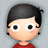#### 学习笔记CB008:词义消歧、有监督、无监督、语义角色标注、信息检索、TF-IDF、隐含语义索引模型

p(s)表达多义词w某个语义s概率，统计大量语料最大似然估计，p(s) = N(s)/N(w)。p(v|s)多义词w某个语义s条件词v概率，p(v|s) = N(v, s)/N(s)。训练出p(s)和p(v|s)，一个多义词w消歧计算(p(c|s)p(s))最大概率。

TF-IDF。TF(term frequency)，一个词在一个文档中出现频率。IDF(inverse document frequency)，一个词出现在多少个文档中。同一个词在短文档出现次数和在长文档中出现次数一样多，对于短文档价值更大。一个出现概率很低的词一旦出现在文档中，价值大于其他普遍出现词。在信息检索领域向量模型做相似度计算非常有效，曾是google发家必杀技。聊天机器人软肋，只考虑独立词，没有任何语义信息。

EM算法，按照最大似然原理，先随便拍一个分布参数，根据分布归类到某一部分，根据归类重新统计数目，按照最大似然估计分布参数，再重新归类、调参、估计，最终得出最优解。每一个训练数据做归类，p(z|d,w)，先拍一个p(z)、p(d|z)、p(w|z)，p(z|d,w)=p(z)p(d|z)p(w|z)/∑p(z)p(d|z)p(w|z)，分子是一个z，分母是所有z和。p(z|d,w)最大似然估计的概率估计（E过程），对每一个训练样本做归类，根据归类好数据统计出n(d,w)，根据公式p(z) = 1/R ∑n(d,w)p(z|d,w)更新参数。p(d|z)=∑n(d,w)p(z|d,w) / ∑n(d,w)p(z|d,w)，分子是一个d和，分母是所有d和，计算p(d|z)最大似然估计。p(w|z)=∑n(d,w)p(z|d,w) / ∑n(d,w)p(z|d,w)，分子一个w和，分母是所有w和，计算p(w|z)的最大似然估计。重新计算p(z|d,w)，p(z|d,w)=p(z)p(d|z)p(w|z)/∑p(z)p(d|z)p(w|z)。不断重复上面EM过程使对数似然函数最大，L=∑∑n(d, w) log P(d, w)。 通过以上迭代得出最终p(w, d)，词与文档之间相关度，利用相关度做检索。

《Python 自然语言处理》

http://www.shareditor.com/blogshow?blogId=88

http://www.shareditor.com/blogshow?blogId=89

http://www.shareditor.com/blogshow?blogId=90